# 线性回归模型详解(Linear Regression)机器学习 同时被 2 个专栏收录25 篇文章 6 订阅

1. 线性回归(Linear Regression)
2. 逻辑回归（Logistic regressions）
3. 多项式回归(Polynomial Regression)
4. 逐步回归(Step Regression)
5. 岭回归(Ridge Regression)
6. 套索回归(Lasso Regression)
7. 弹性网回归(ElasticNet)

## 线性与非线性

• 线性：两个变量之间的关系一次函数关系的——图象是直线，叫做线性。

注意：线性是指广义的线性，也就是数据与数据之间的关系。

• 非线性：两个变量之间的关系不是一次函数关系的——图象不是直线，叫做非线性。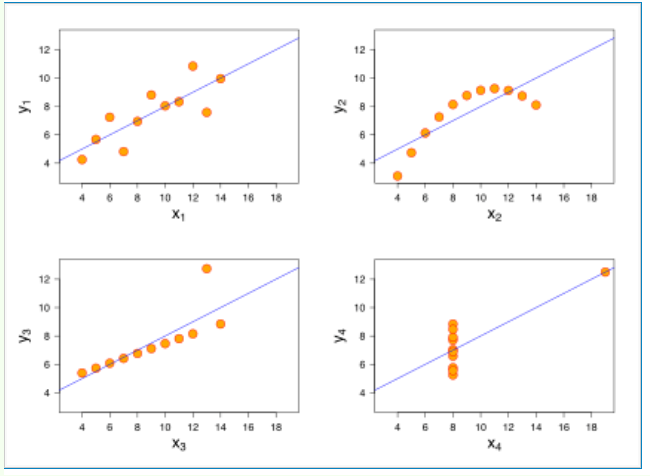• 线性回归是一个回归问题。
• 要预测的变量 y 与自变量 x 的关系是线性的（图2 是一个非线性）。
• 各项误差服从正太分布，均值为0，与 x 同方差（图4 误差不是正太分布）。
• 变量 x 的分布要有变异性。
• 多元线性回归中不同特征之间应该相互独立，避免线性相关。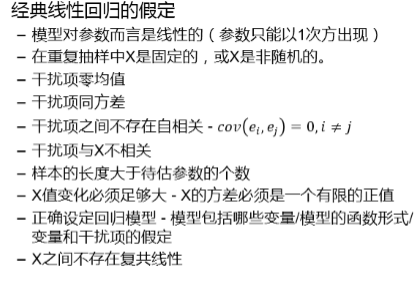## 线性回归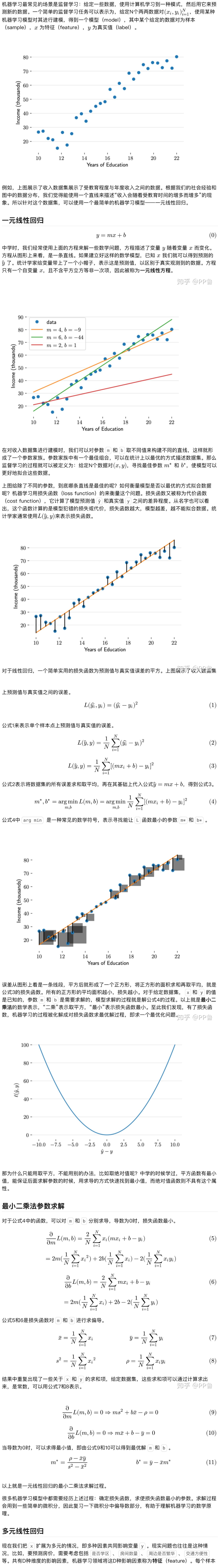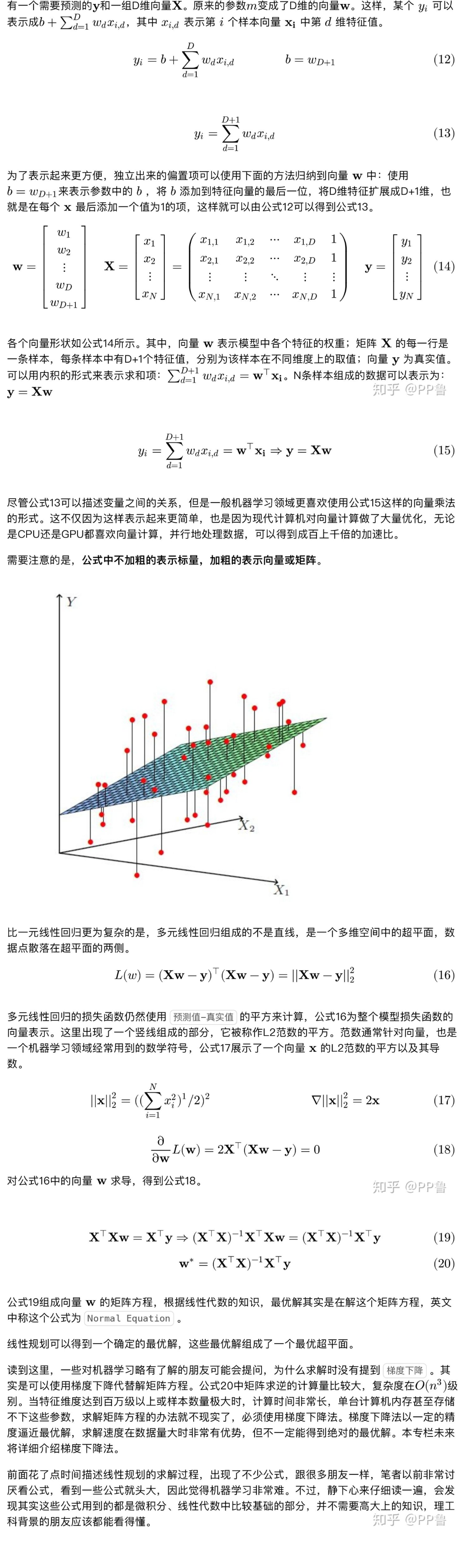## 多重共线性

• 即使预测变量和响应之间存在显著关系，系数也可能看起来并不显著。
• 高度相关的预测变量的系数在样本之间差异很大。
• 从模型中去除任何高度相关的项都将大幅影响其他高度相关项的估计系数。高度相关项的系数甚至会包含错误的符号。

• 数据不足。在某些情况下，收集更多数据可以解决共线性问题。
• 错误地使用虚拟变量。（比如，同时将男、女两个虚拟变量都放入模型，此时必定出现共线性，称为完全共线性）

（1）手动移除出共线性的变量

（2）逐步回归法

（3）增加样本容量

（4）岭回归

## 常用的回归模型评估指标

• 解释方差（ Explained variance score）
• 绝对平均误差（Mean absolute error）
• 均方误差（Mean squared error）
• 决定系数（R² score）

## 算法优缺点

优点：

（1）思想简单，实现容易。建模迅速，对于小数据量、简单的关系很有效；

（2）是许多强大的非线性模型的基础。

（3）线性回归模型十分容易理解，结果具有很好的可解释性，有利于决策分析。

（4）蕴含机器学习中的很多重要思想。

（5）能解决回归问题。

缺点：

（1）对于非线性数据或者数据特征间具有相关性多项式回归难以建模.

（2）难以很好地表达高度复杂的数据。

## 算法实现

import numpy as np
import matplotlib.pyplot as plt

x=np.array([1,2,3,4,5],dtype=np.float)
y=np.array([1,3.0,2,3,5])
plt.scatter(x,y)

x_mean=np.mean(x)
y_mean=np.mean(y)
num=0.0
d=0.0
for x_i,y_i in zip(x,y):
num+=(x_i-x_mean)*(y_i-y_mean)
d+=(x_i-x_mean)**2
a=num/d
b=y_mean-a*x_mean
y_hat=a*x+b

plt.figure(2)
plt.scatter(x,y)
plt.plot(x,y_hat,c='r')
x_predict=4.8
y_predict=a*x_predict+b
print(y_predict)
plt.scatter(x_predict,y_predict,c='b',marker='+')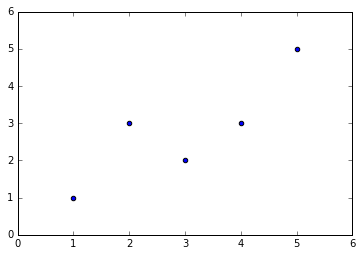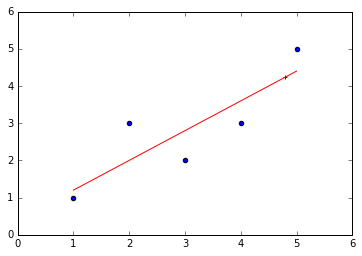import numpy as np
import matplotlib.pyplot as plt
from sklearn.linear_model import LinearRegression  # 线性回归

# 样本数据集，第一列为x，第二列为y，在x和y之间建立回归模型
data=[
[0.067732,3.176513],[0.427810,3.816464],[0.995731,4.550095],[0.738336,4.256571],[0.981083,4.560815],
[0.526171,3.929515],[0.378887,3.526170],[0.033859,3.156393],[0.132791,3.110301],[0.138306,3.149813],
[0.247809,3.476346],[0.648270,4.119688],[0.731209,4.282233],[0.236833,3.486582],[0.969788,4.655492],
[0.607492,3.965162],[0.358622,3.514900],[0.147846,3.125947],[0.637820,4.094115],[0.230372,3.476039],
[0.070237,3.210610],[0.067154,3.190612],[0.925577,4.631504],[0.717733,4.295890],[0.015371,3.085028],
[0.335070,3.448080],[0.040486,3.167440],[0.212575,3.364266],[0.617218,3.993482],[0.541196,3.891471]
]

#生成X和y矩阵
dataMat = np.array(data)
X = dataMat[:,0:1]   # 变量x
y = dataMat[:,1]   #变量y

# ========线性回归========
model = LinearRegression(copy_X=True, fit_intercept=True, n_jobs=1, normalize=False)
model.fit(X, y)   # 线性回归建模
print('系数矩阵:\n',model.coef_)
print('线性回归模型:\n',model)
# 使用模型预测
predicted = model.predict(X)

plt.scatter(X, y, marker='x')
plt.plot(X, predicted,c='r')

plt.xlabel("x")
plt.ylabel("y")

系数矩阵:
[ 1.6314263]
线性回归模型:
LinearRegression(copy_X=True, fit_intercept=True, n_jobs=1, normalize=False)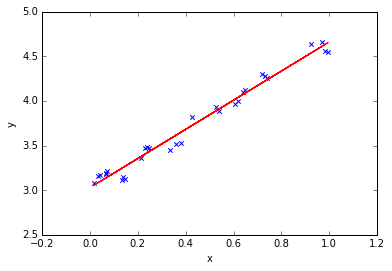04-1215万+11-25444
04-044万+
08-2913万+
08-285万+
06-012万+
07-173万+
10-083万+
08-091407
05-161万+
01-192万+
03-072665
05-216785
07-093697
01-301856
10-144704
03-191301
04-286万+点击重新获取扫码支付余额充值